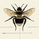# FREE INDICATOR: Laguerre Moving Average by TheLark

15863 views
The Laguerre Average was discovered by John Ehlers .
It's a newer type of averaging that is meant to take out as much of the inherent lag that your typical EMA and SMA's give at the start of a major trend change.
So what you get is an average that turns more quickly at major trend changes, and doesn't get tripped up on the noise (as much).

Usage:
Simply use this in place of EMA or SMA averages, and integrate with your trading style!

By changing the gamma, you change the "length", play with it to see how the average reacts to different inputs, find something you like and run with it!
You can turn off the trend change dots if desired.

Grab the code here: http://pastebin.com/kiMNGrkZ

·´¯`·.¸¸.·´¯`· Feel free to follow me to keep up with my latest scripts! ·´¯`·.¸¸.·´¯`·
·´¯`·.¸¸.·´¯`· PLEASE THUMB UP OR STAR IF YOU LIKE THIS INDICATOR! ·´¯`·.¸¸.·´¯`·
I'd like as many people as possible to get it :)
```study(title = "TheLark: Laguerre Moving Average", shorttitle="TheLark LMA", overlay=true)

//•/•/•/•/•/•/•/•/•/•/•/•/•/•/•/•/•/•/•/•/•/•/•//
//                                             //
//            LAGUERRE MA BY THELARK           //
//                 ~ 2-11-14 ~                 //
//                                             //
//                     •/•                     //
//                                             //
//                                             //
//•/•/•/•/•/•/•/•/•/•/•/•/•/•/•/•/•/•/•/•/•/•/•//

// The Laguerre Average was discovered by John Ehlers.
// It's a newer type of averaging that is meant to take out as much of the
// inherent lag that your typical EMA and SMA's give at the start of a major trend change.
// So what you get is an average that turns more quickly at major trend changes,
// and doesn't get tripped up on the noise (as much).

//setups
h = high
l = low
//inputs
Gamma = input(0.77)
sd = input(true, title="Show dots?")
//calc
lag(g) =>
p = (h + l)/2
L0 = (1 - g)*p+g*nz(L0)
L1 = -g*L0+nz(L0)+g*nz(L1)
L2 = -g*L1+nz(L1)+g*nz(L2)
L3 = -g*L2+nz(L2)+g*nz(L3)
f = (L0 + 2*L1 + 2*L2 + L3)/6
f
//plots
lma = lag(Gamma)
col =  lma > lma ? #0094FF : #FF3571
up = lma > lma ? 1 : 0
down = lma < lma ? 1 : 0
plot(lma,linewidth=2,color=col)
plot(sd and cross(up,down) ? lma : na,style=circles, linewidth=4, color=col )```